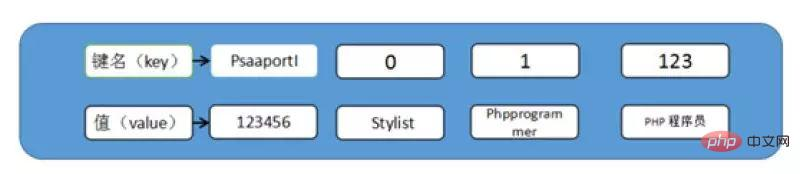# php标量数据和数组的最大区别是什么php标量数据和数组的区别

• 最大区别：一个标量只能存放一个数据，而数组可以存放多个数据。

• 其他区别：标量类型是值传递，而数组是引用传递。

## 什么是标量数据

boolean（布尔型） 最简单的数据类型，只有两个值：true(真) / false(假)
string（字符串） 字符串是连续的字符序列
integer（整型） 整型数据类型包含所有的整数，其可以是整数也可以是负数
float（浮点型） 浮点型数据类型也是用来存储数字，与整型不同它包含小数

1）布尔型（boolean）

`// 代码： \$a = true; // 真值 \$b = false; // 假值`

2）字符串（string）

a.单引号（'）

`\$a = 'zZ爱吃菜';`

b.双引号（"）

`\$b = "zZ爱吃菜";`

`\$a = 'hello'; \$b = '\$a china'; \$c = "\$a world"; // 个人建议这样写：\$c = "{\$a} world"; 不容易产生歧义 echo \$b; echo \$c; 结果： \$a china hello world`

c.定界符（<<<）

1.PHP 定界符的作用就是按照原样，包括换行格式什么的，输出在其内部的东西；
2.PHP 定界符中字符串内容不需要转义

`// 定义 <<<Eof  ……  Eof;`

`\$name = 'kitty'; echo <<<Eof <table height="20"> <tr><td> {\$name}<br/> <script> var p='hello world'; document.writeln(p); </script> </td></tr> </table> <select> <option>aaaaa</option> <option>aaaaa</option> <option>aaaaa</option> </select> Eof;`

3）整型（integer）

`\$int1 = 1234; \$int2 = 01234; \$int3 = 0x1234;  echo "十进制的结果是：{\$int1}<br>"; echo "八进制的结果是：{\$int2}<br>"; echo "十六进制的结果是：{\$int3}<br>"; 结果 十进制的结果是：1234 八进制的结果是：668 十六进制的结果是：4660`

4）浮点型（float）

`// 定义 \$a = 1.036; \$b = 2.035; \$c = 3.48E2; // En代表10*n, E1 代表 * 10, \$c = 348  echo \$c; 结果：348`

## 什么是数组

PHP 数组比其他高级语言中的数组更加灵活，不但支持以数字为键名的索引数组，而且支持以字符串或字符串、数字混合为键名的关联数组。而在其他高级语言中，如 Java 或者 C++ 等语言的数组，只支持数字索引数组。

PHP 数组的结构如下图所示：`<?php echo "<pre>"; class SampleClass { var \$value; } \$a = new SampleClass(); \$a->value = \$a;  \$b = new SampleClass(); \$b->value = &\$b;  echo serialize(\$a); echo "n"; echo serialize(\$b); echo "n"; echo "</pre>"; ?>`

`O:11:"SampleClass":1:{s:5:"value";r:1;} O:11:"SampleClass":1:{s:5:"value";R:1;}`

`echo "<pre>"; class SampleClass { var \$value; } \$a = new SampleClass(); \$a->value = \$a; \$b = new SampleClass(); \$b->value = &\$b; \$a->value = 1; \$b->value = 1; var_dump(\$a); var_dump(\$b); echo "</pre>";`

`object(SampleClass)#1 (1) {    ["value"]=>    int(1) } int(1)`